Courses

# Test: Linear Algebra - 10

## 20 Questions MCQ Test Topic-wise Tests & Solved Examples for IIT JAM Mathematics | Test: Linear Algebra - 10

Description
This mock test of Test: Linear Algebra - 10 for Mathematics helps you for every Mathematics entrance exam. This contains 20 Multiple Choice Questions for Mathematics Test: Linear Algebra - 10 (mcq) to study with solutions a complete question bank. The solved questions answers in this Test: Linear Algebra - 10 quiz give you a good mix of easy questions and tough questions. Mathematics students definitely take this Test: Linear Algebra - 10 exercise for a better result in the exam. You can find other Test: Linear Algebra - 10 extra questions, long questions & short questions for Mathematics on EduRev as well by searching above.
QUESTION: 1

### Let {v1, v2, ........,v16} be an ordered basis for V= C16. If T is a linear transformation on V defined by T(v) = vi+1 for 1 ≤ i ≤ 15 and T(v16) = -(v1 + v2 + ... + v16) Then,

Solution:

Let B = {v1, v2, ........,v16} be an ordered basis for V= C16.
If T is a linear transformation on V defined by T(v) = vi+1 for 1 ≤ i ≤ 15 and T(v16) = -(v1 + v2 + .......+ v16).
Thus, the matrix of T relative to the ordered basis B = {v1, v2, ........,v16} is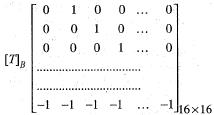Then Rank [TB]= 16
Hence, T is invertible and it has rational eigen values.

QUESTION: 2

### Let T : R3 --> R3 be a linear transformation defined by T(x, y, z) =(x + y - z, x + y + z, y - z) Then, the matrix of the linear transformation T with respect to the ordered basis B = {(0,1,0), (0,0,1), (1,0, 0)| of R3 is

Solution:

Let T : R3 ---> R3 be a linear transformation defined by
T(x,y,z) = (x + y - z , x + y + z, y - z)
We need to find the matrix of T with respect to the ordered basis B = {(0, 1,0), (0,0, 1), (1, 0,0)} of R3.
Now
T(0,1,0) = (1,1,1)
= 1(0, 1, 0) + 1(0, 0, 1) + 1(0, 0, 0)
T(0, 0, 1) = (-1, 1, -1)
= 1(0, 1, 0 ) - 1(0, 0 , 1) - 1( 1, 0 , 0)
T(1, 0, 0) =(1,1,0)
= 1(0,1,0) + 0(0,0,1) + 1(1,0,0)
Therefore, the matrix of T with respect to the ordered basis
B = {(0, 1, 0), (0, 0, 1), (1, 0, 0)} is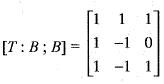QUESTION: 3

### Let T : R4 --> R4 be the linear map satisfying T{e1) =e2, T(e2) = e3, T(e3) = 0, T(e4) = e3, where {e1 e2, e3 e4} is the standard basis of R4. Then,

Solution:

We are given that a linear map
T : R4 --> R4 satisfying
T(e1) = e2, T(e2) = e3, T(e3) - 0, T(e4) = e3
Where {e1 e2, e3, e4} is the standard basis of R4.
Now, let (x,y,z,t) t ∈ R4.
Then (x,y,z,t) = [xe1 + ye2 + ze3 + te4]
Taking the image under linear transformation T, we get
T(x, y, z, t) = T[xe1 + ye2 + ze3 + te4] Using the linearity of T, we get
T(x, y, z, t) = xT(e1) + yT(e2) + zT(e3) + tT(e4)
Substituting the value of T(e1), T(e2), T(e3) and T(e4),
we get
T(x, y, z, t) = xe2 + ye3 + z • 0 + t • e3
or equivalently
T(x, y, z,t) = (0 ,x ,y + t,0)
Let (x, y, z, t) ∈ ker T.
Then T(x, y, z, t) = (0, 0, 0, 0)
Using the definition of linear transformation T, we get
(0, x , y + t, 0) = (0, 0, 0, 0)
Comparing the components, we get
x =0, y + t = 0, z is arbitrary.
Therefore, ker T = {(0, y, z, -y ) : yz ∈ R}.
Hence,dim ker T = 2.
Using rank nullity theorem
Rank T = 4 - dim ker T
= 4 - 2 = 2.
Now T2(x,y,z,t) = T[T(x, y, z, t)]
= T(0, x , y + t, 0) = (0, 0, x, 0)
and T3(x, y, z, t) = T[T2(x, y, z, t)]
= T(0,0,x,0)
= (0,0, 0 ,0 )
Hence, T is nilpotent linear transformation of index 3.

QUESTION: 4

Consider the basis {u1 u2, u3} of R3, where u1 = (1, 0, 0), u2 = (1,1,0) , u= (1,1,1) . Let {f1, f2, f3} be the dual basis of {u1, u2, u3} and f be a linear functional defined by f(a,b,c) = a + b + c, (a, b, c) ∈ R3. If f = a1f1 + a2f2 + a3f3 then (α1, α2, α3) is

Solution:

Let {u1, u2, u3) = {(1, 0, 0), (1, 1, 0), (1,1, 1)}
be the basis o f R3 and {f1, f 2, f3} be the dual basis of {u1, u2, u3} a n d f is a linear functional defined by f(a,b,c) = a + b + c for all (a, b, c) ∈ R3.
Also given that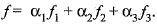Since {f1 + f2 + f3} is dual basis of { u+ u2 + u3}.
Therefore,
f1(u1) = 1, f1(u2) = 0, f1(u3) = 0
f2(u1) = 0 , f3(u2) = l , f2(u3) = 0
f3(u1) = 0 , f3(u2) = 0 , f3(u3) = 1
Let(a,b,c) = xu1 +yu2 + zu3
Substituting th e values of u1, u2 and u3, we get
(a, b, c) = x( 1 ,0 , 0) + y (1,1, 0 ) + z (l, 1 ,1 ) = ( x + y + z , y + z ,z)
Comparing the components of the coordinates, we get
a = x + y + z, b = y + z, c = z
Solving for x, y, z, we get
x = a - b , y = b - c , z = c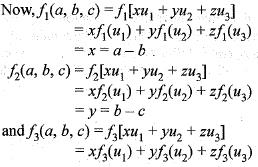= z = c.
Hence, {a - b , b - c , c} is the dual basis o f {u1,u2, u3).
Now since we are given that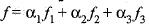we get,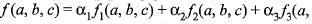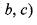or equivalently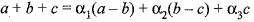or equivalently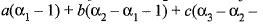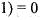C om paring th e coefficients o f a, b, c, we get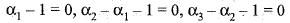Solving for α1, α2, α3 ,we get
α1=1 α=2     α=3

QUESTION: 5

For a matrix [M] =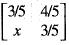, the transpose of the matrix is equal to the inverse of the matrix [M]' = |M| 1 The value of x is

Solution:
QUESTION: 6

The diagonal elements of Skew-Hermitian matrix are

Solution:
QUESTION: 7

The matrix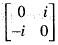is a

Solution:

Explanation : Given a matrix is equal to its conjugate transpose hence it is hermitian. also, it is skew also because the transpose of a given matrix is equal to negative of the given matrix.

Hence it is a skew-Hermitian matrix

QUESTION: 8

If A is a non-zero column vector (n x 1), then the rank of matrix ,AA' is

Solution:
QUESTION: 9

If P and Q are non-singular matrices, then for matrix M, which of the following is correct?

Solution:
QUESTION: 10

Rank of singular matrix of order 4 can be at most

Solution:
QUESTION: 11

If the rank of an n x n matrix A is (n - 1), then the system of equations Ax= b has

Solution:
QUESTION: 12

Let M be a m x n ( m < n) matrix with rank m, then

Solution:
QUESTION: 13

Let M =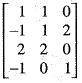then the rank of M is equal to

Solution:
QUESTION: 14

Let A be a matrix of order m x n and R is non-singular matrix of order n, then

Solution:
QUESTION: 15

Let A be a square matrix of order n, then nullity of A is

Solution:
QUESTION: 16

A is a 3 x 4 real matrix and AX = b is an inconsistent system of equations. The highest possible rank of A is

Solution:
QUESTION: 17

The system of linear equations 4x + 2y =7, 2x + y = 6 has

Solution:
QUESTION: 18

If x + 2y - 2u = 0, 2x - y - u = 0, x + 2z - u = 0, 4x -y + 3 z - u= 0 is a system of equations, then it is

Solution:
QUESTION: 19

Let T : P3[0 ,1] --> P2[0 , 1] be defined by (Tp) (x) = P"(x) + P'(x). Then the matrix representation of T with respect to the basis {1, x, x2, x3} and {1, x, x2} of P3[0, 1] and P2[0, 1], respectively is

Solution:

Let T : P3[0, 1] --> P2[0,1] be a linear transformation defined by (Tp) (x) = p"(x) + p'(x ). We need to find the matrix of T with respect to the basis { l , x , x2, x3} and {1, x, x2} of P3[0, 1] and P2[0, 1] respectively.
Now,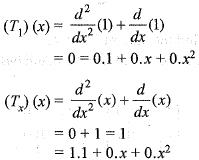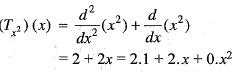and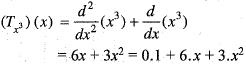Therefore, the matrix of T with respect to the basis {1, x, x2, x3} and { 1,x,x2} of P3[0, 1] and P2[0, 1] respectively is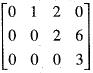QUESTION: 20

Let T : R4 —> R4 be defined by
T(x, y , z, w) = (x + y + 5w, x + 2 y + w, - y - z + 2w, 5x + y+ 2z).
Then dimension of the eigen space of T is

Solution:

We are given th at a linear transform ation T : R4 —> R4 defined by
T(x, y, z, w) = x + y + 5w, x + 2y + w, w - z + 2w, 5x + y + 2z)
Let B = {(1, 0, 0, 0), (0, 1, 0, 0), (0, 0 , 1 , 0), (0, 0, 0 ,1 ) } be standard basis of R4. Then
T(l, 0 ,0 ,0 ) = (1,1, 0,5) = 1( 1, 0, 0, 0) + 1(0, 1, 0, 0) + 0(0, 0, 1, 0) + 5(0, 0, 0, 1)

T(0, 1 ,0 ,0 ) = ( 1 ,2 ,0 , 1)
= 1( 1, 0, 0, 0) + 2(0 , 1, 0, 0) + 0(0,
0, 1, 0) + 1(0, 0, 0, 1)

T(0, 0, 1, 0) = (0, 0, - 1 , 2 )
= 0 (1 ,0 , 0, 0) + 0 ( 0 , 1 ,0 , 0 ) - l (0, 0, 1, 0) + 2(0, 0, 0, 1)
T(0, 0, 1) = (5, 1, 2, 0) = 5(1, 0,0 ,0 )+ 1 (0 , 1,0,0)+ 2 (0, 0, 1, 0) + 0(0,0,0,1)
Therefore, the matrix of linear transformation T with respect to the basis B is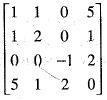which is a
symmetric matrix and hence diagonalisable.
Hence, dimension of eigen space is 4

Track your progress, build streaks, highlight & save important lessons and more!

### Similar Content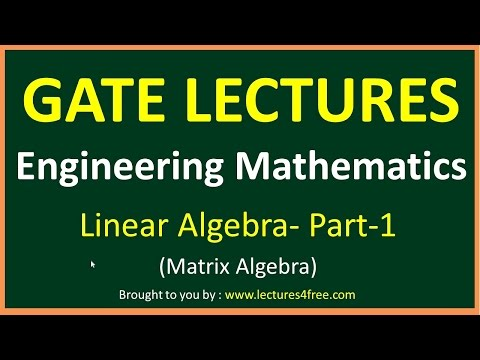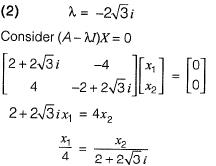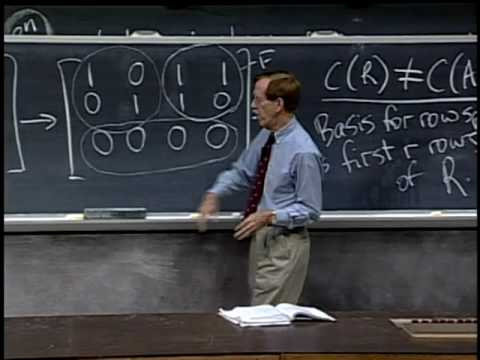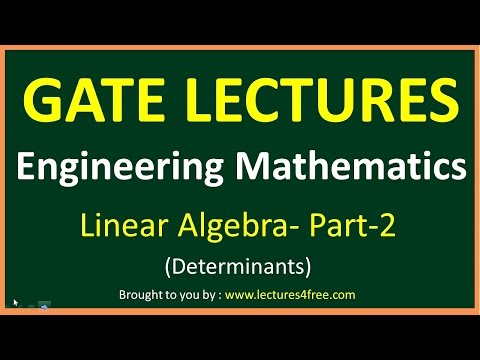### Related tests